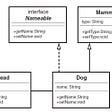# Solving in JS. Base 3 to Integer

## We are given this question.

`Given a string s representing a number in base 3 (consisting only of 0, 1, or 2), return its decimal integer equivalent. This should be implemented directly without using a built-in function.let s = "121" //output = 16`

We all heard of the concept of base 10 or the decimal. For example 1, 10, 100, 1000 and so forth. It is called base 10 because it consists of only(0, 1, 2, 3, 4, 5, 6, 7 ,8, or 9) 10 possible numbers.

We also all have heard of the binary number (bi means 2), which are 0 and 1. It is a computer language, each string represents a specific character. For example “1100001” will represent the number 97. Then we can take that number and convert it into a readable human language, 97 equals the lower case alphabet “a”.

`let a = 97console.log(a.toString(2))// "1100001"let b = "1100001"console.log(parseInt(b, 2))// 97let c = String.fromCharCode(97)console.log(c)// "a"let d = "a".charCodeAt(0)console.log(d)// 97`

## Visual Representation Example

Base 10 — includes (0,1,2,3,4,5,6,7,8,9)

`Let n = "13852"Base 10 raise to the power of the position give us the value for that column.Then we multiple the value by the number of times to get the sum of that column.When we add all the column together we get the integer sum.10,000 + 3,000 + 800 + 50 + 2 = 13,852"13852" ==> 13,852`

Base 2 — includes(0,1)

`Let n = "11001"Base 2 raise to the power of the position give us the value for that column.Then we multiple the value by the number of times to get the sum of that column.When we add all the column together we get the integer sum.16 + 8 + 0 + 0 + 1 = 25"11001" ==> 25`

## Back to our main challenge, base 3.

Using what we know about base, the same idea applies to base 3. Which consist of only (0, 1, or 2), hence 3 numbers.

`function base3(s) {    let sum = 0    for(let i = s.length -1; i >= 0; i--){        if(Number(s[i]) !== 0){            let position = s.length -1 - i            sum += Number(s[i]) * (3 ** position)        }    }    return sum}`
1. First we create a sum variable with the value 0. Update the sum as we loop through the string number.
2. When we read, it’s left to right with index 0 being on the most left. When we add, it’s right to left with the index 0 starting at the most right. In our code, `let i = s.length -1 `to get the last index of the string number. Transverse the string number till we reach index 0.
3. To get the current position (index) of the column we need to subtract the current index from the last index. `let position = s.length -1 — i`
4. To get the value of that column, raise the base of 3 to the position.
5. To get the sum of the column, multiple the value with the number of `s[i]`
6. The sum then get added to the running sum variable that we setup initially.
7. We can wrap the code in a if statement to check if `Number(s[i])` its not 0 because 0 + 0 is still 0, so we skip over that iteration.
`base3("121") // 16`

--

--

--

## More from Dong Xia

Copy and Paste what’s on my mind. Perfect.

Love podcasts or audiobooks? Learn on the go with our new app.

## If you’re a developer, beware of this npm package## NodeJS on App Engine Standard## Active Record and the Wildcard: A Tale of More Than One Search Term## Is React Native even worth it?## A Guide to Learn React.Js## Introducing BLoC Pattern with React and RxJS## Dong Xia

Copy and Paste what’s on my mind. Perfect.

## How to Fix the ERR_SSL_PINNED_KEY_NOT_IN_CERT_CHAIN error?## Creating Object Models with UML## Functions in MATLAB: Finding the minimum of a function (Part-2)